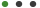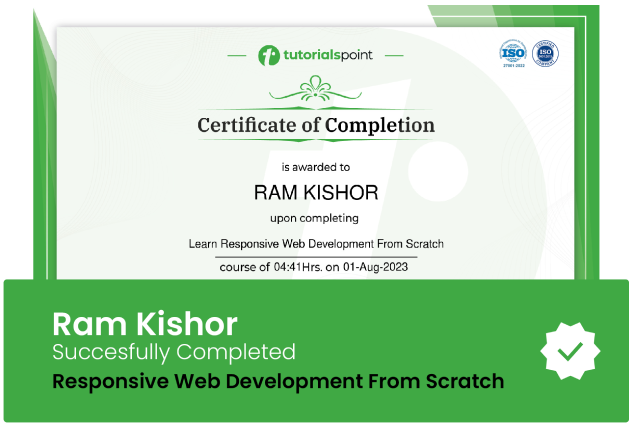### Mathematics for Data ScienceErmin Dedic

# Mathematics for Data Science

obtain all the neccesary math knowledge required for data scienceUpdated on Sep, 2023Language - EnglishErmin DedicData Science and AI ML,Math,Data & Analytics30-days Money-Back Guarantee

Training 5 or more people ?

## Course Description

Ermin presents the material through an interactive whiteboard presentation.

The course starts with Linear Algebra.

We start with a definition of what a linear equation is, look at forms of a linear equation, define systems of linear equations, consider notation, and how to solve systems of equations via Row Echelon Form (REF) and Reduced Row Echelon Form (R-REF), and perform matrix-vector multiplication. Then, we explore the concept of mathematical structures to better understand the idea of a vector space, before dealing with concepts like subspaces, bases for vector spaces, dimensions of a vector space/subspace, linear maps, orthogonal projection, and how that is related to least-squares approximation.

The next section is an intro to probability. You will first explore the idea of probability models and axioms, simple counting, before considering discrete cases of marginal probability, conditional probability, and Bayesian probability. You will also discover the concept of independence and permutations and combinations. Next, the idea of a random variable is illustrated, along with the probability mass and density function, cumulative distribution function, covariance/correlation, the law of large numbers, and central limit theorem. In the final part, you will discover statistical inference. You will see how the Bayesian Estimator works.

### Goals

What will you learn in this course:

• Define and Solve a System of Linear Equations
• Describe the concept of a Vector Space and Subspace
• Discuss the concepts of linear combinations, span, and basis confidently.
• Identify the idea of a Probability Model and its Axioms
• Indicate the purpose of a random variable
• Compare and contrast a Probability Mass Function and Probability Density Function
• Compute a Joint PDF
• Recall what the Law of Large Numbers and Central Limit Theorem tell us
• Estimate error via Bayesian Estimator

### Prerequisites

What are the prerequisites for this course?

• No prerequisites. This course is geared towards beginners.## Curriculum

Check out the detailed breakdown of what’s inside the course

Intro to Linear Algebra
14 Lectures
•Linear Equation Definition 04:51 04:51
•Forms of a Linear Equation 03:40 03:40
•Systems of Linear Equations 02:56 02:56
•Line and Plane 02:54 02:54
•Aij Notation 05:27 05:27
•System of Equations as a Matrix 04:50 04:50
•System in Corresponding Forms 07:40 07:40
•Row Echelon Form (Gaussian Elimination) 06:43 06:43
•Reduced Row Echelon Form 04:21 04:21
•Row Operations Example (REF) 09:07 09:07
•Row Operations Rules 05:41 05:41
•Visualizing Ax=b 03:23 03:23
•General Formula - Matrix Vector Multiplication 09:15 09:15
•Tips for Row Operations 06:47 06:47
Mathematical Structures
17 LecturesIntro to Probability
8 LecturesRandom Variables and Multiple Discrete and Continuous Variables
12 LecturesStatistical Inference
4 Lectures## Instructor DetailsErmin Dedic

All Things Data.

I have a passion for anything data, whether it is applying statistical methods to data more generally, or utilizing a data-driven approach in the Healthcare or Finance/Banking industries.

I studied Psychology for 6-years, including 2 years of Graduate school, where I was training to be a Child/School Psychologist. I had an opportunity to experience a blend of course work and clinical work but also recognize some of the problems facing the mental health system and graduate school system.

While I did ultimately decide to voluntarily leave the Grad program, it is via academics that I fell in love with statistics and statistical software like SPSS/SAS.

It was my Graduate school experience that solidified my interest in teaching, and where I received a lot of great feedback on my teaching and teaching style.

## Course Certificate

User your certification to make a career change or to advance in your current career. Salaries are among the highest in the world.